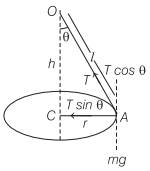# Examples of Centripetal Force in Everyday Life – Motion in a Plane

## Examples of Centripetal Force in Everyday Life – Motion in a Plane

We are giving a detailed and clear sheet on all Physics Notes that are very useful to understand the Basic Physics Concepts.

### Centripetal Force Examples in Real Life

If centripetal force is obtained only by the force of friction between the tyres of the vehicle and road, then for a safe turn, the coefficient of friction (ug) between the road and tyres should be
$$\mu_{s} \geq \frac{v^{2}}{r g} \text { or } \quad v \leq \sqrt{\mu_{s} r g}$$
where, v is the velocity of the vehicle and r is the radius of the circular path.
Maximum velocity for no skidding or slipping is vmax = $$\sqrt{\mu r g}$$
If centripetal force is obtained only by the banking of roads, then the speed (v) of the vehicle for a safe turn
$$v=\sqrt{r g \tan \theta}$$

If speed of the vehicle is less than $$\sqrt{r g \tan \theta}$$, then it will move inward (down) and r will decrease and if speed is more than $$\sqrt{r g \tan \theta}$$, then it will move outward (up) and r will increase.

In normal life, the centripetal force is obtained by the friction force between the road and tyres as well as by the banking of the roads.

Therefore, the maximum permissible speed for the vehicle is much greater than the optimum value of the speed on a banked road.

When centripetal force is obtained from friction force as well as banking of roads, then maximum safe value of speed of vehicle
$$v_{\max }=\sqrt{\frac{r g\left(\tan \theta+\mu_{s}\right)}{\left(1-\mu_{s} \tan \theta\right)}}$$

Bending of Cyclist:
When a cyclist takes turn at road, he inclines himself from the vertical, slows down his speed and moves on a circular path of larger radius.
If a cyclist is inclined at an angle θ, then tan θ = $$\frac{v^{2}}{r g}$$
where,
v = speed of the cyclist
r = radius of path and g = acceleration due to gravity.

Conical Pendulum Time Period:
It consists of a string OA whose upper-end O is fixed and bob is tied at the other free end. The string is whirled in a horizontal circle, then the arrangement is called a conical pendulum.Angular speed, ω = $$\frac{v}{r}=\sqrt{\frac{g \tan \theta}{r}}$$
Time period of conical pendulum, T = $$2 \pi \sqrt{\frac{l \cos \theta}{g}}$$

Death Well or Rotor:
In this, a person drives a bicycle on a vertical surface of large wooden well, while in case of a rotor at a certain angular speed of rotor a person hangs resting against the wall without any support from the bottom.
In both the cases, Safe speed, v = $$\sqrt{\frac{g r}{\mu}}$$

Motion in a Plane (Projectile and Circular Motion):
In this chapter or under this topic, we are going to come across the motion of the object when it is thrown from one end to another end. This practice is said to be projection. Also, when an object is moved in a circular motion, then the equation of the motion is derived here. We will learn here about centripetal force and centripetal acceleration in detail with formulas. Also learn the force applied in everyday life motion of the particle in a vertical circle.